GeeksforGeeks App
Open AppBrowser
Continue

## Related Articles

Limiting friction is defined as the friction which is generated between the two static surfaces that come in contact with each other. The maximum friction that can be generated between two static surfaces in contact with each other. Once a force applied to the two surfaces exceeds the limiting friction, the motion will occur.

For two dry surfaces, the limiting friction is a product of the normal reaction force and the coefficient of limiting friction. In other words, the amount of friction that can be applied between two surfaces is limited and if the forces acting on the body are made sufficiently great, the motion will occur.

Hence, we can define limiting friction as the maximum value of static friction that comes into play when the body is just at the point of sliding over the surface of another body.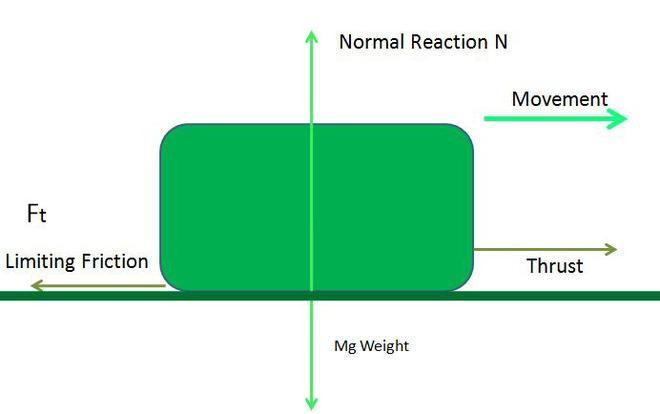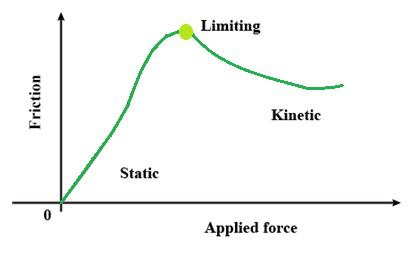### Laws of Limiting Friction

Following are the laws of limiting friction:

(a) The direction limiting frictional force and the direction of motion is always the opposite.

(b) The limiting friction always acts tangential to the two surfaces that are in contact.

(c) The magnitude of the limiting friction and the normal force between the two surfaces are directly proportional.

(d) The limiting friction is dependent on the material, the nature of the surfaces that are in contact, and the smoothness.

(e) The limiting friction is independent of the shape and area of the surfaces.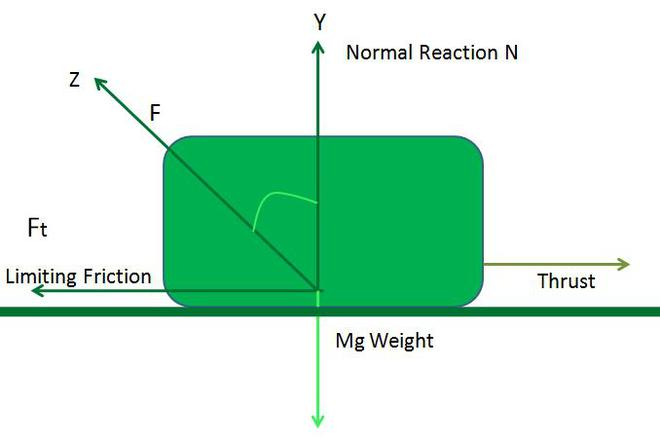### Examples of Limiting Friction

Following are some of the examples of Limiting Friction:

1. When we walk on the road.
2. When a car moves on a cliff.
3. Friction force applied by upstream water to a swimmer.
4. Friction force on a pen by a page or notebook.
5. Moving an object on the ground.
6. A car parked on a hill will not slide down. This is because the value of limiting friction is high enough to ensure that the car is in a state of rest. The limiting force of friction is directly proportional to the mass of the object.
7. Pushing a heavy truck seems very difficult due to the high value of static friction
8. When we push any object we encounter static friction for some time until the value of limiting friction is reached.

### Formula and its Derivation

Hence, we can define limiting friction as the maximum value of static friction that comes into play when the body is just at the point of sliding over the surface of another body. Limiting friction is the product of normal force and the coefficient of limiting friction.

Following is the mathematical representation:

Friction force applied to the body is always proportional to the normal force acting on the body.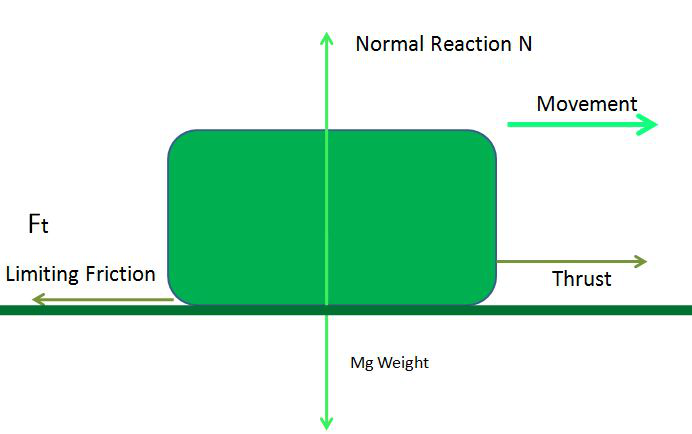To remove the proportion sign we introduce a constant.

Now,Where,

F is the limiting frictionis the coefficient of limiting friction

N is the normal force

Example: Find the normal force applied on a body having a coefficient of friction of 5 and limiting friction is 50 N.

Solution:

As we knowWhere,

F is the limiting frictionis the coefficient of limiting friction

N is the normal forceN = 10 N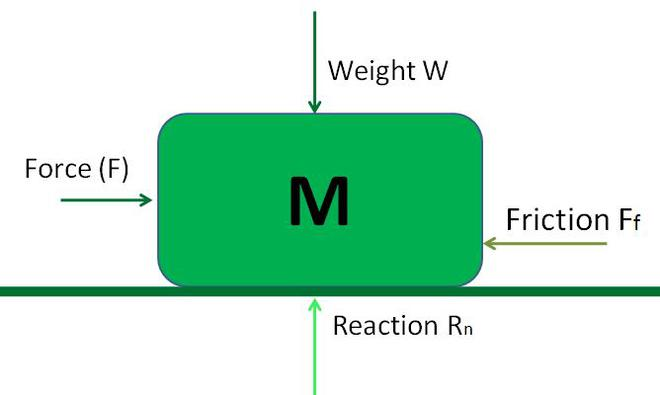### Sample Questions

Question 1: What is the other name for limiting friction?

It is the maximum value of friction. Thus limiting friction is also called as maximum value of friction.

Question 2: What is the difference between static friction and limiting friction?

Static friction is a self-adjusting force because it comes into play when the body is lying over the surface of another body without any motion. When that body overcomes the force of static friction, the maximum value of static friction is reached, which is known as limiting friction.

Question 3: What is the relationship between kinetic and limiting friction?

When the applied force, F, is increased further (beyond the limiting frictional force), the body begins to move, then the force opposing the motion is called the kinetic or sliding friction. The kinetic friction is less than the limiting friction.

Question 4: Why is kinetic friction less than limiting friction?

Initially it requires more force to break the interlock between the irregularities of two surfaces. But once the block is in motion, it takes time to interlock between the irregularities of two surfaces. Also, the body gains the inertia of motion. So, the kinetic friction is less than the limiting friction.

Question 5: Is static or limiting friction greater?

In short, static friction is the same as the force being applied because the body isn’t moving.Static friction is acting due to the body tends to move when a force is acting on it.Limiting friction is the friction acting on a body when it is about to start moving.Commonly, limiting friction is the highest.

Question 6: Is limiting friction a variable?

When that body overcomes the force of static friction, the maximum value of static friction is reached -which is known as limiting friction. After the limiting friction, the frictional force is not going to increase further. At this stage, the object moves overcoming the frictional force which is at a constant value.

### Numerical Problems

Question 1. Find the minimum force required to move a 5 kg box if the coefficient of friction is 0.2.

Solution:

Minimum force is nothing but the value of friction force due to frictional surface because if we apply force more than friction force then box can be move.

N = m×g

N = 5kg × 10m/s2 = 10 N

F = μ × N

F = 0.2 × 10 = 2 N

So, we require minimum 2N to move the box.

Question 2. If the minimum force required to move a table is 100 N then find the weight of the table when μ = 5.

Solution:

Minimum force required to move a table (F) =\mu \times N

Given, F = 100 N and \mu = 5Question 3. Find coefficient of limiting friction if 100 kg of Machine requires 10000 N force to change the position.()

Solution:

F = 10000 N

M = 1000 kg

N = m × g N = 100 kg × 10m/s2 N = 1000 N F = μ × N 10000 N = μ × 1000 N μ = 10

My Personal Notes arrow_drop_up
Related Tutorials